wingyuen kan

•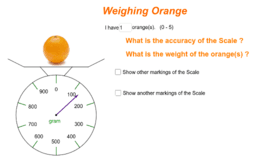Error in measurement 2 -- weighing orange

Activity

wingyuen kan

•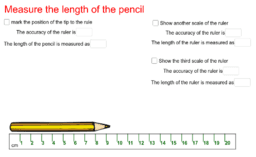Error in measurement 1 -- the length of pencil

Activity

wingyuen kan

•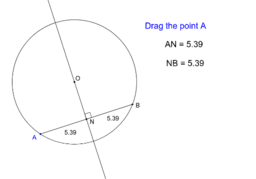The Geometry of Circle

Book

wingyuen kan

•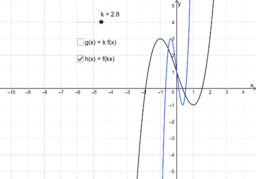Enlargement of Function

Activity

wingyuen kan

•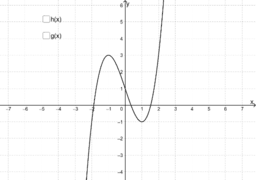Reflection of Function

Activity

wingyuen kan

•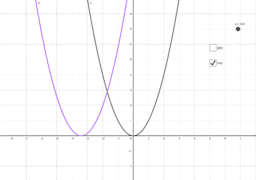Translation of Function

Activity

wingyuen kan

•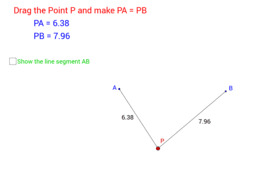Locus

Book

wingyuen kan

•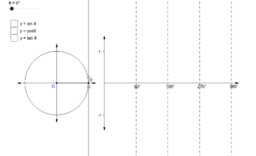Graphs of Trigonometric Functions

Activity

wingyuen kan

•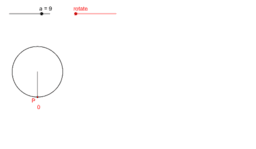Exploration of the circumference of circle

Activity

wingyuen kan

•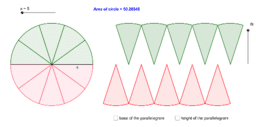Exploration of the area of circle

Activity

wingyuen kan

•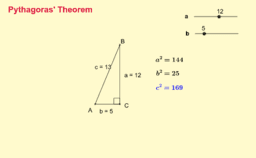Explorating Pythagoras' Theorem

Activity

wingyuen kan

•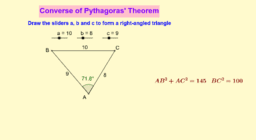Converse of Pythagoras' Theorem

Activity

wingyuen kan

•Perpendicular from Centre Bisects Chord

Activity

wingyuen kan

•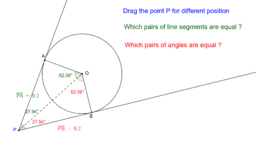Tangent from External Point

Activity

wingyuen kan

•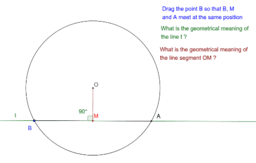Activity

wingyuen kan

•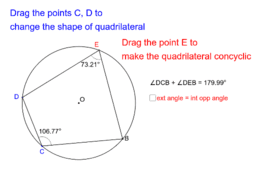Test for Concyclic points (2)

Activity

wingyuen kan

•Test for Concyclic Points (1)

Activity

wingyuen kan

•Activity

wingyuen kan

•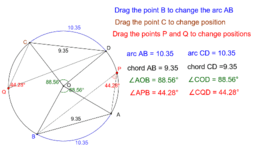Equal Arcs Equal Chords Equal Angles

Activity

wingyuen kan

•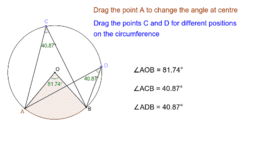Angles in the Same Segment

Activity

wingyuen kan

•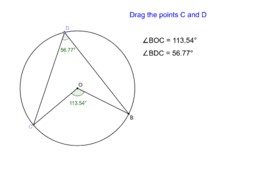Angle at Centre and Angle at Circumference

Activity

wingyuen kan

•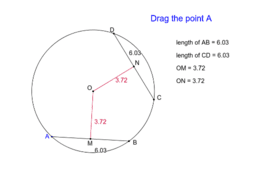Equal Chord Equal Distance from Centre

Activity

wingyuen kan

•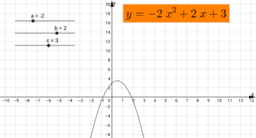Exploring the features of the graph of a quadratic function

Activity

wingyuen kan

•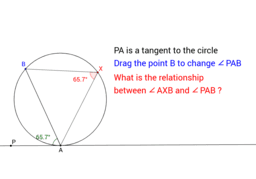Activity

wingyuen kan